Formatted question description: https://leetcode.ca/all/1516.html

# 1516. Move Sub-Tree of N-Ary Tree

Hard

## Description

Given the root of an N-ary tree of unique values, and two nodes of the tree p and q.

You should move the subtree of the node p to become a direct child of node q. If p is already a direct child of q, don’t change anything. Node p must be the last child in the children list of node q.

Return the root of the tree after adjusting it.

There are 3 cases for nodes p and q:

1. Node q is in the sub-tree of node p.
2. Node p is in the sub-tree of node q.
3. Neither node p is in the sub-tree of node q nor node q is in the sub-tree of node p.

In cases 2 and 3, you just need to move p (with its sub-tree) to be a child of q, but in case 1 the tree may be disconnected, thus you need to reconnect the tree again. Please read the examples carefully before solving this problem.

Nary-Tree input serialization is represented in their level order traversal, each group of children is separated by the null value (See examples).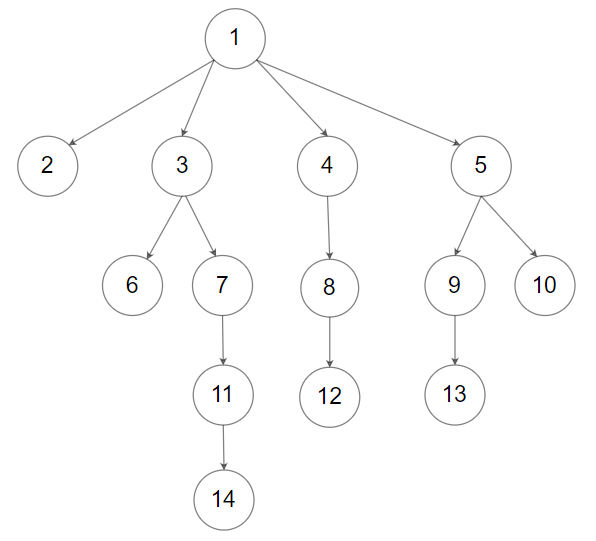For example, the above tree is serialized as [1,null,2,3,4,5,null,null,6,7,null,8,null,9,10,null,null,11,null,12,null,13,null,null,14].

Example 1: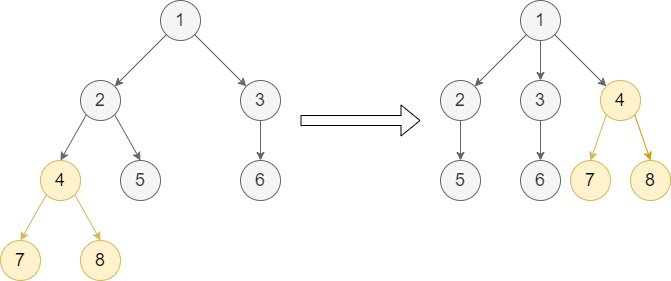Input: root = [1,null,2,3,null,4,5,null,6,null,7,8], p = 4, q = 1

Output: [1,null,2,3,4,null,5,null,6,null,7,8]

Explanation: This example follows the second case as node p is in the sub-tree of node q. We move node p with its sub-tree to be a direct child of node q.

Notice that node 4 is the last child of node 1.

Example 2: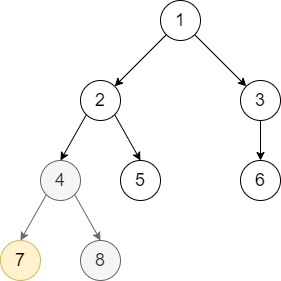Input: root = [1,null,2,3,null,4,5,null,6,null,7,8], p = 7, q = 4

Output: [1,null,2,3,null,4,5,null,6,null,7,8]

Explanation: Node 7 is already a direct child of node 4. We don’t change anything.

Example 3: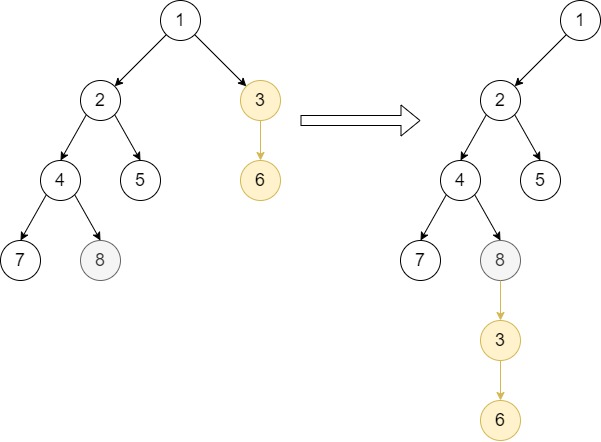Input: root = [1,null,2,3,null,4,5,null,6,null,7,8], p = 3, q = 8

Output: [1,null,2,null,4,5,null,7,8,null,null,null,3,null,6]

Explanation: This example follows case 3 because node p is not in the sub-tree of node q and vice-versa. We can move node 3 with its sub-tree and make it as node 8’s child.

Example 4: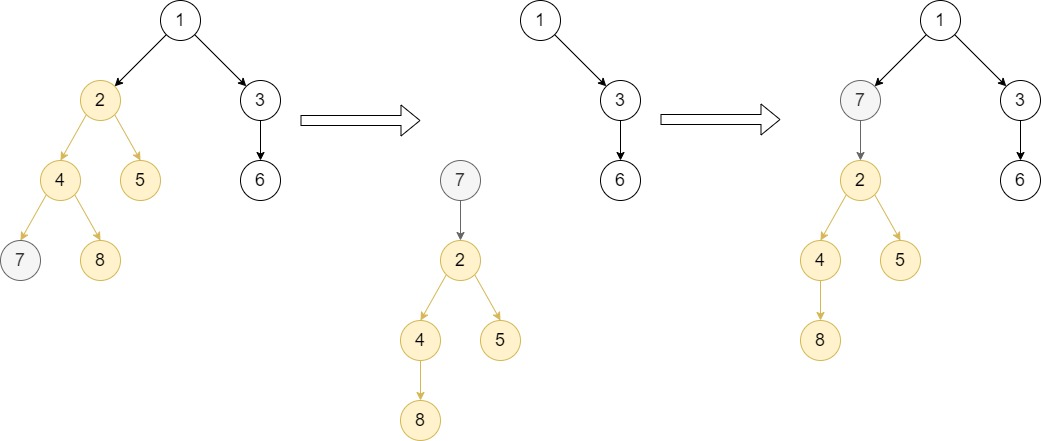Input: root = [1,null,2,3,null,4,5,null,6,null,7,8], p = 2, q = 7

Output: [1,null,7,3,null,2,null,6,null,4,5,null,null,8]

Explanation: Node q is in the sub-tree of node p, so this is case 1.

The first step, we move node p (with all of its sub-tree except for node q) and add it as a child to node q.

Then we will see that the tree is disconnected, you need to reconnect node q to replace node p as shown.

Example 5: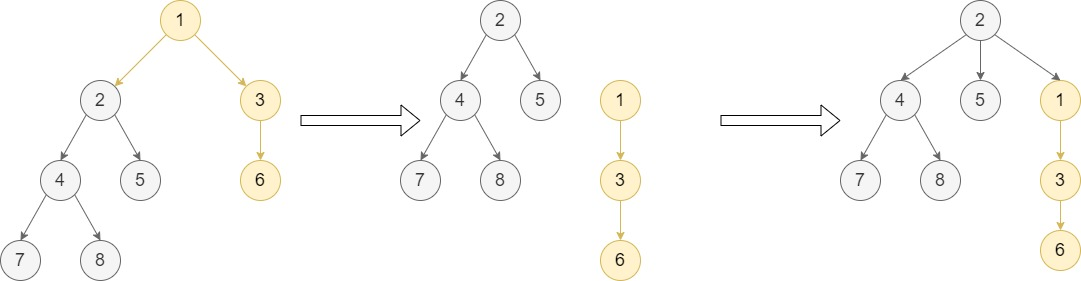Input: root = [1,null,2,3,null,4,5,null,6,null,7,8], p = 1, q = 2

Output: [2,null,4,5,1,null,7,8,null,null,3,null,null,null,6]

Explanation: Node q is in the sub-tree of node p, so this is case 1. The first step, we move node p (with all of its sub-tree except for node q) and add it as a child to node q.

As node p was the root of the tree, node q replaces it and becomes the root of the tree.

Constraints:

• The total number of nodes is between [2, 1000].
• Each node has a unique value.
• p != null
• q != null
• p and q are two different nodes (i.e. p != q).

## Solution

Do depth first search to find each node’s parent. If node q is already the parent of node p, then simply return the original tree.

Otherwise, check whether node q is in the subtree of node p. If node p is not the root of the N-ary tree, then if node q is in the subtree of node p, remove node q from its parent’s children and replace node p with node q in node p’s children. If node q is not in the subtree of node p, remove node p from its parent’s children. Finally add node p to node q’s children, and return the N-ary tree’s root.

If node p is the root of the N-ary tree, then remove node q from its parent’s children, add node p to node q’s children, and return node q as the root of the new N-ary tree.

/*
// Definition for a Node.
class Node {
public int val;
public List<Node> children;

public Node() {
children = new ArrayList<Node>();
}

public Node(int _val) {
val = _val;
children = new ArrayList<Node>();
}

public Node(int _val,ArrayList<Node> _children) {
val = _val;
children = _children;
}
};
*/

class Solution {
public Node moveSubTree(Node root, Node p, Node q) {
Map<Node, Node> parentMap = new HashMap<Node, Node>();
Deque<Node> stack = new LinkedList<Node>();
stack.push(root);
while (!stack.isEmpty()) {
Node node = stack.pop();
List<Node> children = node.children;
for (int i = children.size() - 1; i >= 0; i--) {
Node child = children.get(i);
parentMap.put(child, node);
stack.push(child);
}
}
if (parentMap.get(p) == q)
return root;
boolean flag = false;
Node temp = q;
while (temp != null) {
Node parent = parentMap.get(temp);
if (parent == p) {
flag = true;
break;
} else
temp = parent;
}
if (parentMap.containsKey(p)) {
Node pParent = parentMap.get(p);
if (flag) {
Node qParent = parentMap.get(q);
qParent.children.remove(q);
int index = pParent.children.indexOf(p);
pParent.children.set(index, q);
} else
pParent.children.remove(p);
return root;
} else {
Node qParent = parentMap.get(q);
qParent.children.remove(q);